# Making Big Magic Squares

### A method of combining smaller magic squares to make bigger ones.

By

Elsewhere on this site, I give magic square recipes for making odd sized magic squares, or some even sized magic squares. There's also a java applet that can make magic squares of any small enough size...

On this page, I show how to take two small magic squares to make a much bigger magic square. For example, if you wanted an 18x18 magic square, you could get one by combining a 6x6 magic square and a 3x3 magic square.

I'll illustrate the method using two famous historical magic squares, Lo Shu's 3x3 magic square, and Albrecht Durer's 4x4 magic square. These are illustrated below.Let's call the 4x4 square 'Magic Square A', and the 3x3 magic square 'Magic Square B'. The result is going to be a 12x12 square (since 3 times 4 is 12). The first step is to draw a big 12x12 grid for the final magic square. You'll need four or five copies.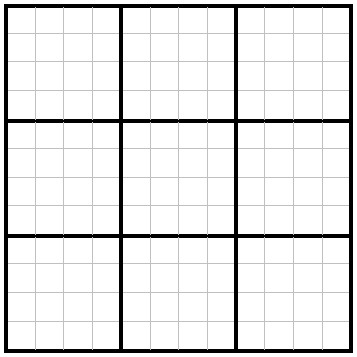Notice that the 12x12 grid is divided into 4x4 blocks - the same size as Magic Square A, and these blocks are arranged in a 3x3 pattern, just like Magic Square B.

Take one of the empty grids, and make lots of copies of Magic Square A, like you see in the picture below.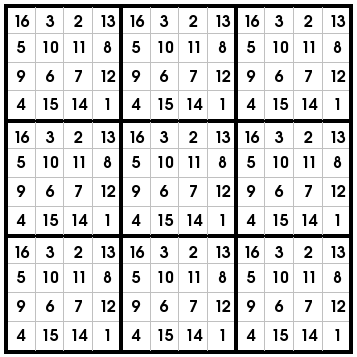Already, this is sort of a magic square, since each row, column or diagonal adds up to 102. However, each number is repeated many times, so we can do better than that.

Leave this grid aside for the moment, and take another copy of the empty grid. Each block corresponds to one cell of Magic Square B. Fill each block with the corresponding number from the magic square, as shown below.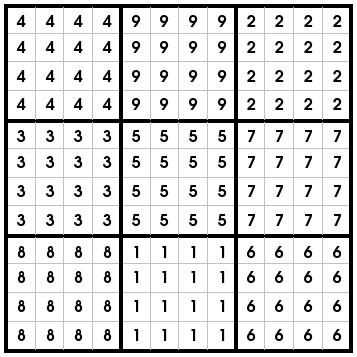Again, this is sort of a magic square - each row, column or diagonal adds up to 60.

To get a proper 12x12 magic square, using the numbers 1 to 144 each exactly once, we have to do some arithmetic. First, take the second big grid, and do the following steps :

• Subtract one from each cell (so the numbers are from 0 to 8 instead of 1 to 9).
• Then, multiply each cell by 16 (because each number is repeated 16 times)
When I do that with my example, I get this :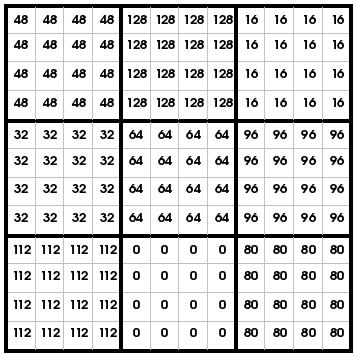To build our final 12x12 magic square, take this grid, the first big grid, and a new empty grid. Then, add together the numbers in corresponding cells.

• For example, looking at the top left cell, my first grid shows 16, my last one shows 48. Adding these, I get 64. So, I write 64 in the top left cell of the new empty grid.
• For the next cell, the first grid shows 3, the last one shows 48. Adding these gives 51, so I write 51 in the next cell of the big grid.
• I keep going until I've filled in all 144 cells of the empty grid. The result is shown below.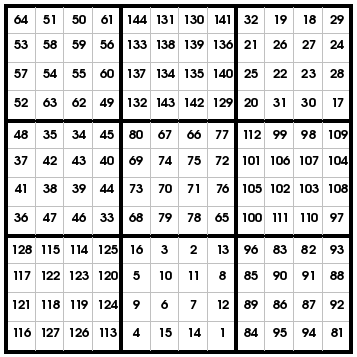In the end (unless I make a mistake!) I have a 12x12 grid containing each of the numbers from 1 to 144 exactly once. Each row, column and diagonal adds up to the same number (870). In other words, I have a magic square!

### How can this be used for teaching?

Obviously, it takes a lot of arithmetic to get the result. Since there are no 2x2 magic squares (and using the 1x1 magic square doesn't lead to anything very interesting), the smallest possible magic square you could make using this method would be a 9x9 magic square (by combining two 3x3 magic squares). You'll have to judge whether your class can handle the amount of arithmetic needed - however, it might be ideal for an advanced class that's excited about magic squares.

On the other hand, this method might make a perfect programming exercise for students learning how to program computers...

Hope that helps!